## A cylinder with moment of inertia I1 rotates with angular speed ω0 about a frictionless vertical axle. A second cylinder, with moment of ine

Question

A cylinder with moment of inertia I1 rotates with angular speed ω0 about a frictionless vertical axle. A second cylinder, with moment of inertia I , initially not rotating, drops onto the first cylinder. Since the surfaces are rough, the two eventually reach the same angular speed ωf.

a. Calculate ωf. (Use I1 for I1, I2 for I2, and omega_i for ωi, as necessary.)

b. Show that the kinetic energy of the system decreases in this interaction by calculating the ratio of the final to initial rotational energy. Express your answer in terms of ωi.

in progress 0
5 months 2021-08-24T22:01:00+00:00 1 Answers 2 views 0

Part(a): The final angular velocity is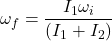Part(b): The ratio of the rotational energies is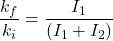,showing the the energy of th system will decrease.

Explanation:

Part(a):

If ‘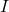‘ be the moment of inertia of an object and ‘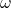‘ be its angular velocity then the angular momentum ‘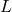‘ of the object can  be written as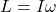If ‘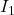‘ and ‘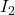‘ be the moment of inertia of the two cylinders and ‘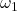‘ and ‘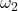‘ be the initial angular velocity of the cylinders and ‘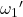‘ and ‘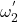‘ be their respective final angular velocity, then from conservation of angular momentum,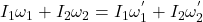Given,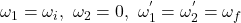. From the above expressionPart(b):

Initial kinetic energy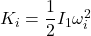and Final kinetic energy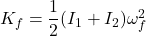Substituting the value of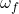,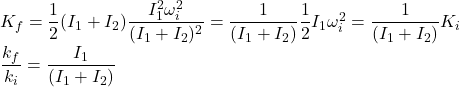The above expression shows that the ebergy of the system will decrease.